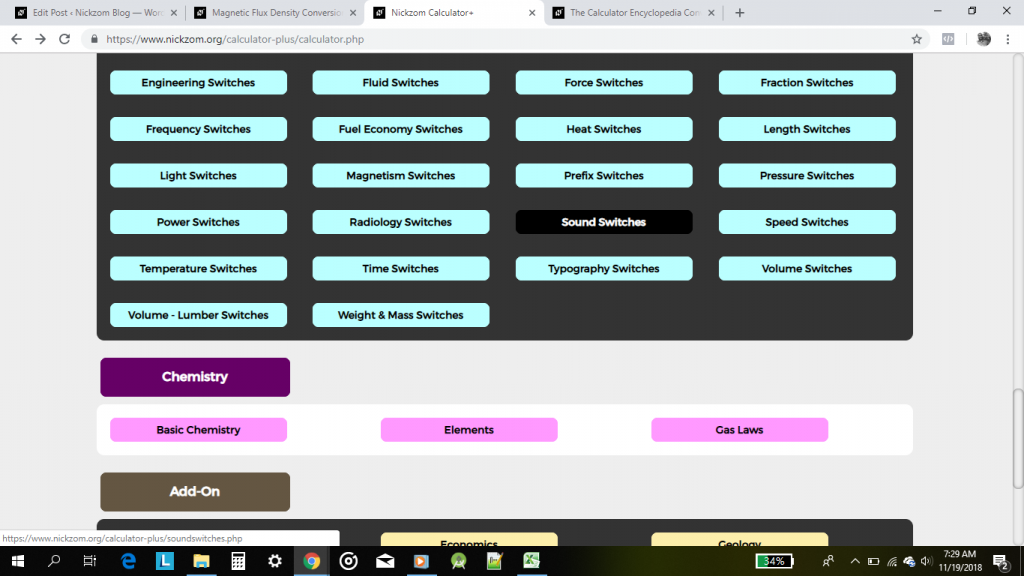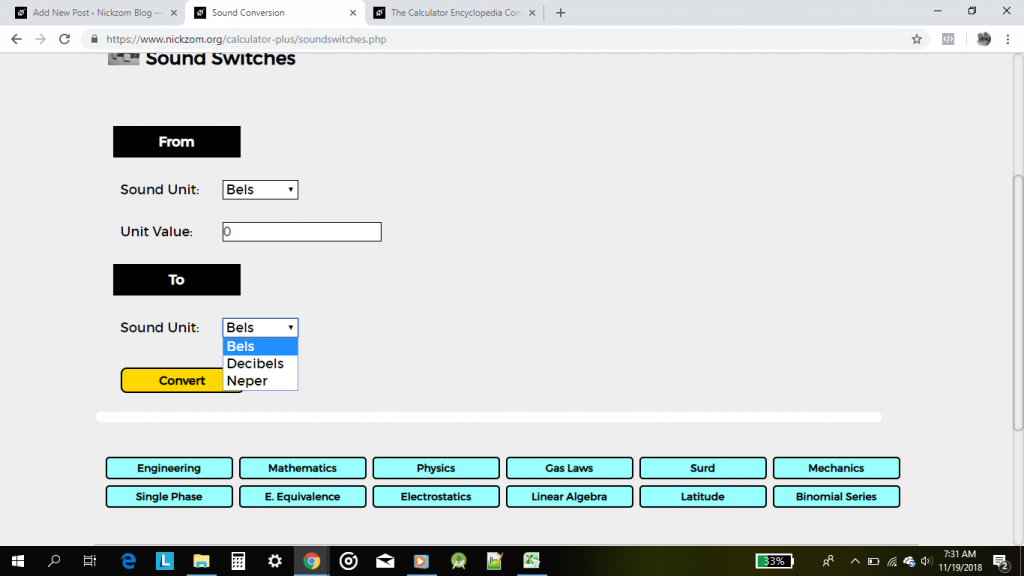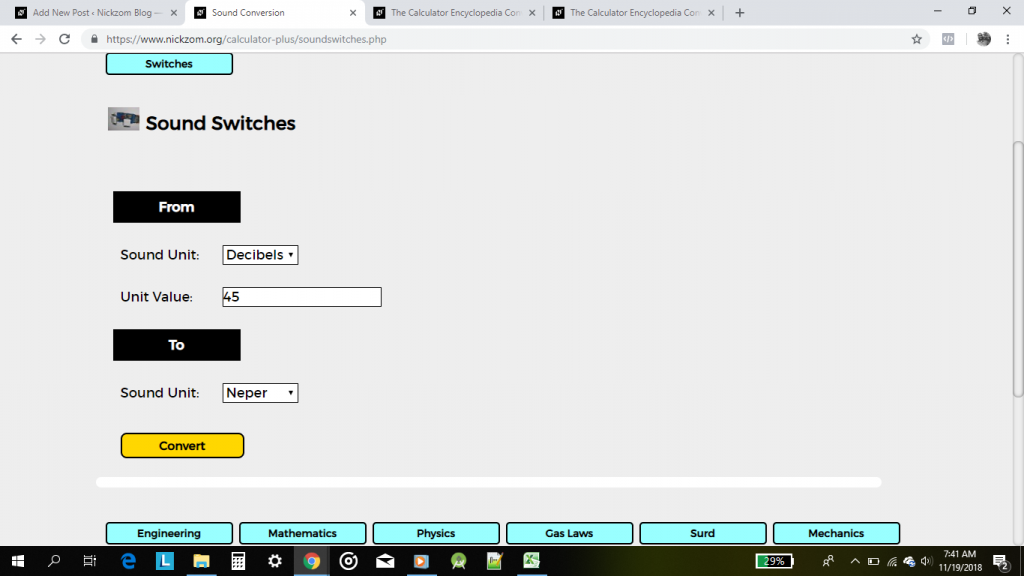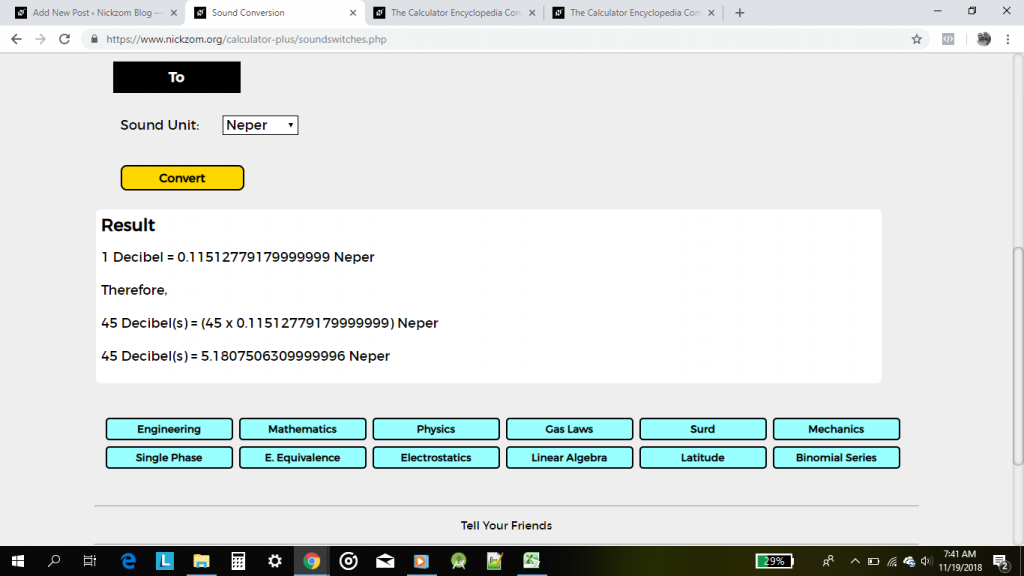# The Calculator Encyclopedia Converts Units of Sound

According to Wikipedia,

The decibel (symbol: dB) is a unit of measurement used to express the ratio of one value of a physical property to another on a logarithmic scale. It can be used to express a change in value (e.g., +1 dB or −1 dB) or an absolute value. In the latter case, it expresses the ratio of a value to a reference value; when used in this way, the decibel symbol should be appended with a suffix that indicates the reference value, or some other property. For example, if the reference value is 1 volt, then the suffix is “V” (e.g., “20 dBV”), and if the reference value is one milliwatt, then the suffix is “m” (e.g., “20 dBm”).

Also, according to Wikipedia,

The neper (symbol: Np) is a logarithmic unit for ratios of measurements of physical field and power quantities, such as gain and loss of electronic signals. The unit’s name is derived from the name of John Napier, the inventor of logarithms. As is the case for the decibel and bel, the neper is a unit defined in the international standard ISO 80000. It is not part of the International System of Units (SI), but is accepted for use alongside the SI.

Nickzom Calculator+ (Professional Version) – The Calculator Encyclopedia converts the units of sounds and at the moment, it converts three of them which are:

• Bels
• Decibels
• NeperClick on Sound SwitchesFor the purpose of this post, we are to convert 45 Decibels to Neper

Now, enter the unit value and select the appropriate units accordinglyLastly, click on Convert buttonOne can access Nickzom Calculator+ (Professional Version) – The Calculator Encyclopedia via any of these channels:

## One thought on “The Calculator Encyclopedia Converts Units of Sound”

1.تسديد قروض says:

Thanks for a marvelous posting! I truly enjoyed reading it, you are a great author.
I will remember to bookmark your blog and will often come
back later on. I want to encourage continue your great writing, have a nice day!